Next: Extended trapezium rule Up: C. Trapezium rule for Previous: C. Trapezium rule for   Contents

## Trapezium rule

The trapezium rule states that you can approximate the area under a curve by a trapezium, as shown in figure C.4. The area is therefore calculated like this: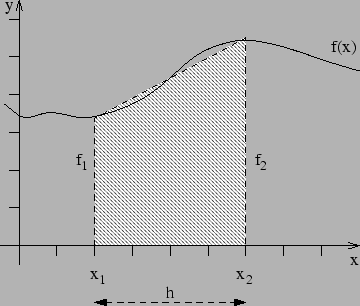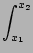f (x)dx = h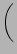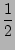f1 +f2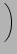+ O(h3f'')

Where the notation f1 is short hand for f (x1).

The error due to the approximation, O(h3f''), indicates that this method is exact for polynomials up to degree 1, i.e. straight lines f (x) = ax + b, because for such functions the second derivative f'' = 0. It is clearly an approximation for higher order polynomials (x2,x3, etc.) and other functions. The error will also be small if h is small.

The area of a trapezium is its average height x its width, i.e.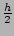(f1 + f2).Next: Extended trapezium rule Up: C. Trapezium rule for Previous: C. Trapezium rule for   Contents
RHUL Dept. of Physics